# RSI Indicator: Stocks, Formula, Calculation and Strategies

The Relative Strength Index (RSI) indicator, as the name suggests, tells us the relative strength of the asset. In other words, the RSI tells us how well the stock is performing (or not) with respect to itself. RSI is counted as a robust technical indicator which can be used to analyse the market and is an important part of the trader’s arsenal as it helps them to make better decisions in timing the market. Of course, like other indicators, it is always advisable to use more than one indicator as it helps us in avoiding the limitations and over dependency on just one.

Thus, in this blog, along with understanding the RSI indicator, we will also look at its limitations and when to use them. We would be covering the following points in this blog article:

If you already know how to find the RSI indicator values, jump to the RSI based strategies here.

### RSI calculation with the help of an example

Let’s understand how to calculate and graph the RSI indicator now. While you can easily calculate the RSI indicator value with the python code, for explanation purposes we will do it manually.

 Date Close (1) Change (2) Gain (3) Loss (4) Avg Gain (5) Avg Loss (6) RS (7) 14-day RSI (8) 24-04 283.46 25-04 280.69 -2.77 0.00 2.77 26-04 285.48 4.79 4.79 0.00 27-04 294.08 8.60 8.60 0.00 30-04 293.90 -0.18 0.00 0.18 01-05 299.92 6.02 6.02 0.00 02-05 301.15 1.23 1.23 0.00 03-05 284.45 -16.70 0.00 16.70 04-05 294.09 9.64 9.64 0.00 07-05 302.77 8.68 8.68 0.00 08-05 301.97 -0.80 0.00 0.80 09-05 306.85 4.88 4.88 0.00 10-05 305.02 -1.83 0.00 1.83 11-05 301.06 -3.96 0.00 3.96 14-05 291.97 -9.09 0.00 9.09 3.13 2.52 1.24 55.37 15-05 284.18 -7.79 0.00 7.79 2.91 2.90 1.00 50.07 16-05 286.48 2.30 2.30 0.00 2.86 2.69 1.06 51.55 17-05 284.54 -1.94 0.00 1.94 2.66 2.64 1.01 50.20 18-05 276.82 -7.72 0.00 7.72 2.47 3.00 0.82 45.14 21-05 284.49 7.67 7.67 0.00 2.84 2.79 1.02 50.48 22-05 275.01 -9.48 0.00 9.48 2.64 3.27 0.81 44.69 23-05 279.07 4.06 4.06 0.00 2.74 3.03 0.90 47.47 24-05 277.85 -1.22 0.00 1.22 2.54 2.90 0.88 46.71 25-05 278.85 1.00 1.00 0.00 2.43 2.70 0.90 47.45 29-05 283.76 4.91 4.91 0.00 2.61 2.50 1.04 51.05 30-05 291.72 7.96 7.96 0.00 2.99 2.32 1.29 56.29 31-05 284.73 -6.99 0.00 6.99 2.78 2.66 1.05 51.12 01-06 291.82 7.09 7.09 0.00 3.09 2.47 1.25 55.58 04-06 296.74 4.92 4.92 0.00 3.22 2.29 1.40 58.41 05-06 291.13 -5.61 0.00 5.61 2.99 2.53 1.18 54.17

#### Step 1: Closing Price

We will take the closing price of the stock for 30 days. The closing price is mentioned in column (1).

#### Step 2: Changes in Closing Price

We then compare the closing price of the current day with the previous day’s closing price and note them down. Thus, from the table, for 25-04, we get the change in price as (280.69 - 283.46) = -2.77.

Similarly, for 26-04, Change in price = (Current closing price - Previous closing price) = (285.48 - 280.6) = 4.79. We will then tabulate the results in the column mentioned as “Change (2)”. In this manner, we calculated the change in price.

#### Step 3: Gain and Loss

We will now create two sections depending on the fact the price increased or decreased, with respect to the previous day’s closing price.

If the price has increased, we note down the difference in the “Gain” column and if it’s a loss, then we note it down in the “Loss” column.

For example, on 26-04, the price had increased by 4.79. Thus, this value would be noted in the “Gain” column.

If you look at the data for 25-04, there was a decrease in the price by 2.77. Now, while the value is written as negative in the “change” column, we do not mention the negative sign in the “Loss” column. And only write it as 2.77. In this manner, the table for the columns “Gain (3)” and “Loss (4)” is updated.

#### Step 4: Average Gain and Loss

In the RSI indicator, to smoothen the price movement, we take an average of the gains (and losses) for a certain period.

While we call it an average, a little explanation would be needed. For the first 14 periods, it is a simple average of the values.

To explain it, we will look at the average gain column.

Thus, in the table, the first 14 values would be from (25-04) to (14-05) which is, (0.00 + 4.79 + 8.60 + 0.00 + 6.02 + 1.23 + 0.00 + 9.64 + 8.68 + 0.00 + 4.88 + 0.00 + 0.00 + 0.00)/14 = 3.13.

Now, since we are placing more emphasis on the recent values, for the next set of values, we use the following formula,

[(Previous avg. gain)*13)+ current gain)]/14.

Thus, for (15-05), we will calculate the average gain as [(3.13*13)+0.00]/14 = 2.91.

Similarly, we will calculate the average Loss too.

Based on these formulae, the table is updated for the columns “Avg Gain (5)” and “Avg Loss (6)”.

#### Step 5: Calculate RS

Now, to make matters simple, we add a column called “RS” which is simply, (Avg Gain)/(Avg Loss). Thus, for 14-05, RS = (Avg Gain)/(Avg Loss) = 3.13/2.52 = 1.24.

In this manner, the table for the column “RS (7)” is updated. In the next step, we finally work out the RSI values.

#### Step 6: Calculation of RSI

RSI = [100 - (100/{1+ RS})].
For example, for (14-05),

RSI = [100 - (100/{1+ RS})] = [100 - (100/{1+ 1.24})] = 55.37.

In this manner, the table is updated.

This is how we get the value of RSI. The RSI indicator graph is always created with respect to the closing price. For example, if we take into account the values of RSI in the above table, the graph should be as follows: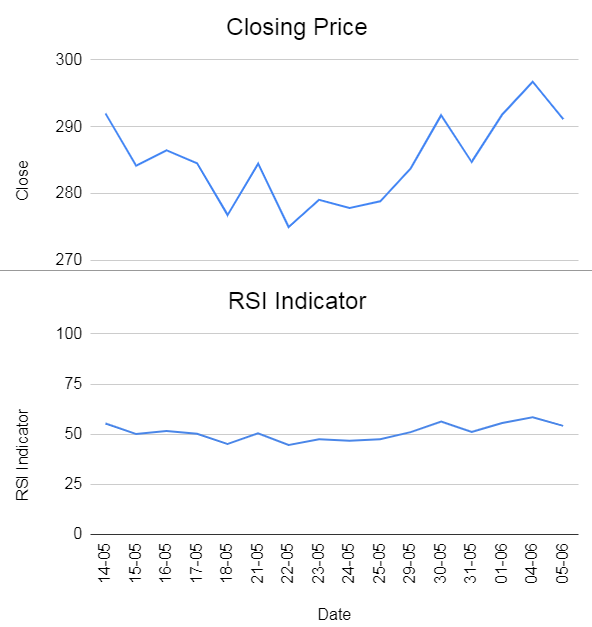While the formula for RSI mentioned here is generally accepted, we can have certain variations on the formula based on the weightage given to the Average gain or loss values. It is a known fact that as the number of data points increase, the RSI Indicator gets better at gauging the market. Certain sources recommend at least 250 data points before you start making trading decisions on them.

One of the reasons why the RSI indicator is popular is because its value is always between 0 and 100. This makes it easy to read and form opinions about the asset. Furthermore, it is generally accepted that a value below 30 indicates “oversold” while a value over 70 indicates “overbought”.

However, it is not as easy as it looks, and if we try to enter and exit the market based on this factor alone, we would not be able to trade effectively as there are numerous occasions where this advice can fail. You can see in the graph below that the closing price continued to rise even after entering the overbought zone from 19 March to 25 April.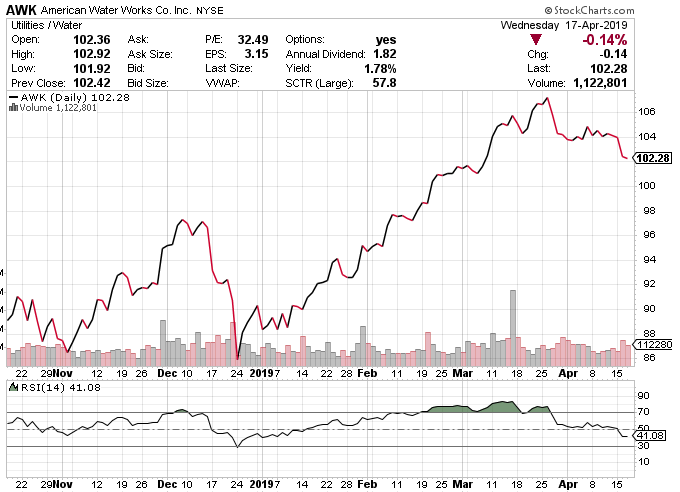With this in mind, let us try to see a few strategies based on the RSI indicator.

### RSI Strategies

#### RSI as a trend indicator

RSI works as a reference when you want to gauge if the market is going through a bullish or bearish trend. While the general assumption that an indicator above 70 indicates overbought and below 30 indicates oversold holds true for most of the cases, there are others who insist that it can be held true for values above 66.6 and below 33.3 as well.

If the RSI closes above 66.6 but goes below the value, it should not be assumed that the trend has reversed and you should short the stock immediately. Instead, we look for the graph and as long as it stays above 33.3, we can say that the market is still bullish when it comes to the asset.

You can see in the graph below that the RSI indicator crossed the overbought zone in July 2018 and since then, the price continued to rise indicating a bull run.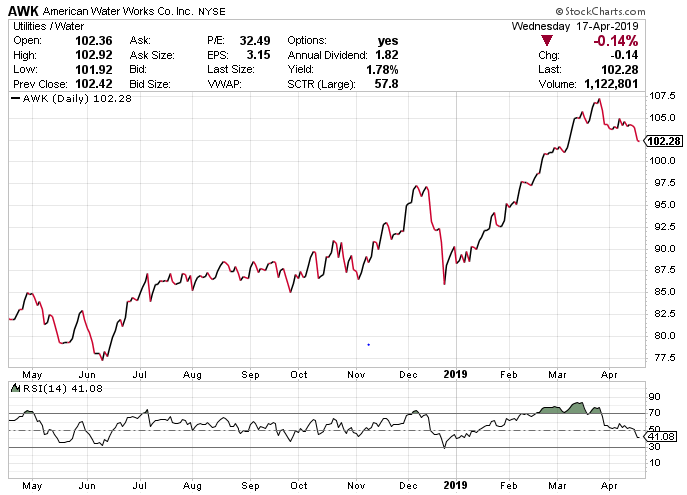Similarly, if the RSI goes below 33.3 and rises up but doesn’t cross the 66.6 barriers (or 70, as is assumed), we can conclude that the market is bearish.

However, one should not take this as a sole indication to buy (or sell) the asset in question. We will go forward to the next topic to understand the whole picture.

#### RSI as support and resistance

As we have understood how to set up the RSI trend indicator, we can see that as the number of periods of gains increases when compared to the number of periods of losses, the RSI values will keep increasing. Thus, it gives a strong indication of a longer-term trend than the closing prices where the daily price fluctuations could show us a different story.

Just like the closing prices, we can use the RSI indicator values to draw a trendline of the support and resistance levels and thus, a breakout from this trend can be easily observed in the RSI values a market position taken. You can see in the following graph how both the RSI and closing price have a breakout.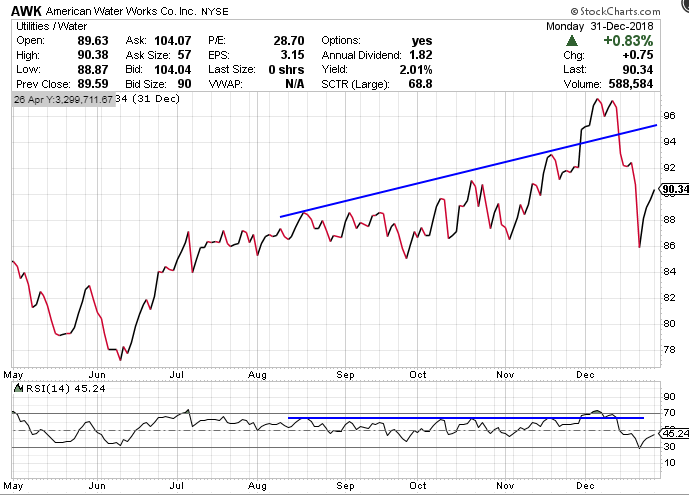The deal here is to correctly identify if the breakout is sustainable for a long period or a false signal.

#### RSI and divergence

RSI indicator can also be used to predict a divergence in the trend before the price trend actually reverses.

Divergence can usually be spotted when, for example, if the price line is moving higher but the RSI indicator slumps due to the fact that the relative strength of the asset weakens when compared to the previous periods’ growth.

For example, let’s say that the price has been closing higher than the previous days but the gain is not as substantial as compared to the average gain of the period. Then, the RSI will close lower than the previous day's value. In this regard, we can get a signal that the market will get a check on the price in a few days and thus trade accordingly. You can see that in the following graph where the RSI shows a downward trend while the prices keep rising. Eventually, the closing prices decrease too.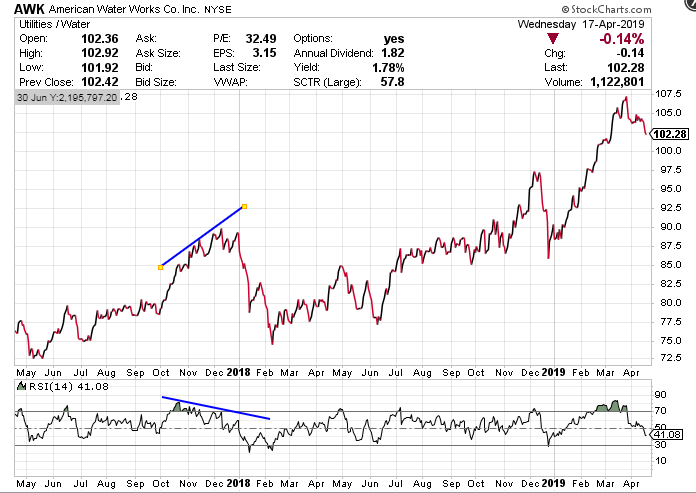The same is true when the closing price has been bearish for a while but the RSI starts posting higher values, it means that the prices will pick up.

#### RSI double bottom signal and double top signal

As we have discussed in the section on Bollinger Bands, we can identify the double bottom and double top pattern using the RSI indicator too. The gist of the double bottom pattern identification is as follows.
1. The RSI indicator closes below 30 before rising higher and above the 30 barriers.
2. It again heads south but closes above 30 before rising again sharply.
This is seen as a “W” formation on the chart. Traders usually use this pattern as a confirmation that the market is headed for the bullish direction. You can see in the following graph that the RSI indicator breached the 30 barriers on 2 Jan before rising up sharply and then closed below, but above the 30 barriers on 7 Jan. It then rose again and continued to rise throughout the month of January and February which indicates a bullish run.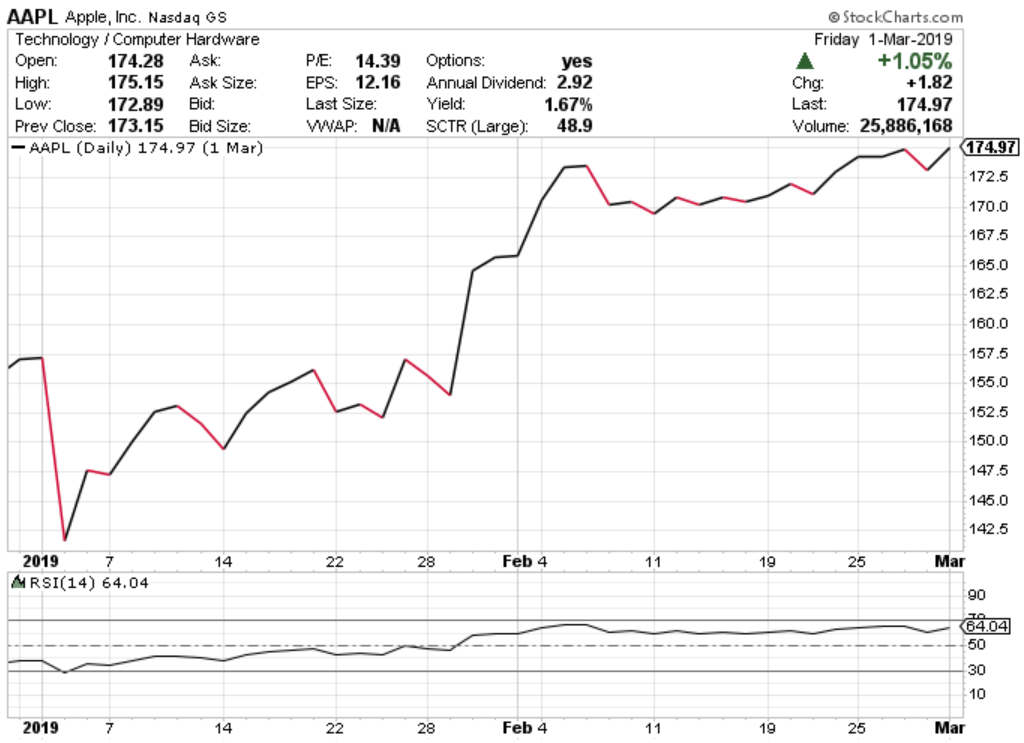Similar to the double bottom, the pattern formation for the double top is as follows:

1. The RSI indicator breaches the 70 levels before dropping again below the 70 levels.
2. It again rises but closes below 70 and then drops sharply again.
This is seen as an “M” formation on the price chart. Traders usually use this pattern as a confirmation that the market is headed for the bearish direction. You can see in the graph given below, the RSI level breaches 70 on Dec '17. Later, it falls below the 70 thresholds and while it does rise higher, it does not cross the 70 thresholds again and then starts dropping, indicating a bearish run which lasts a year.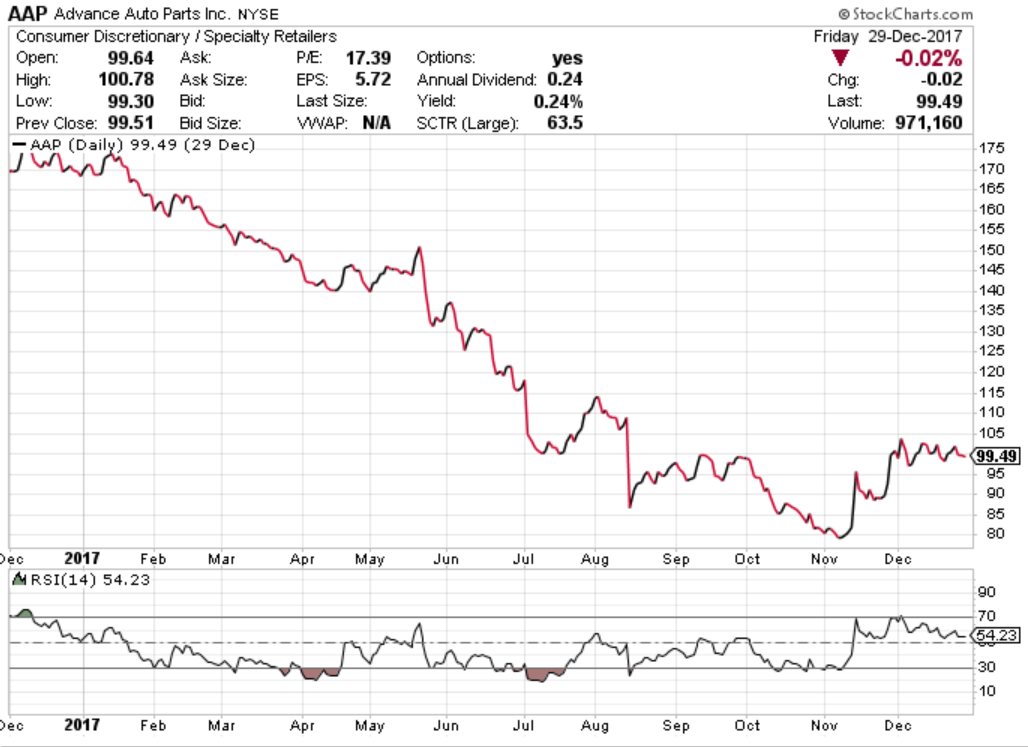#### RSI and MACD strategy

We have long understood that using a sole indicator as the basis for your trading decisions can be disastrous if we fail to read or there is a false signal generated by the indicator. The smart investor uses 2 or more indicators to identify a positive (or negative) signal and enters the market accordingly. With this factor, we use RSI and MACD to identify signals which can be used to trade profitably. To understand MACD and how to calculate it, you can refer to this article.

Why do we use RSI and MACD indicators together?

The reason we use RSI and MACD together is they complement each other due to their inherent nature of comparing the price action of an asset.

In this strategy, we enter the trade when both the RSI and MACD indicate that the asset is oversold and exit when either of the indicators gives us a signal that the price has entered the overbought zone.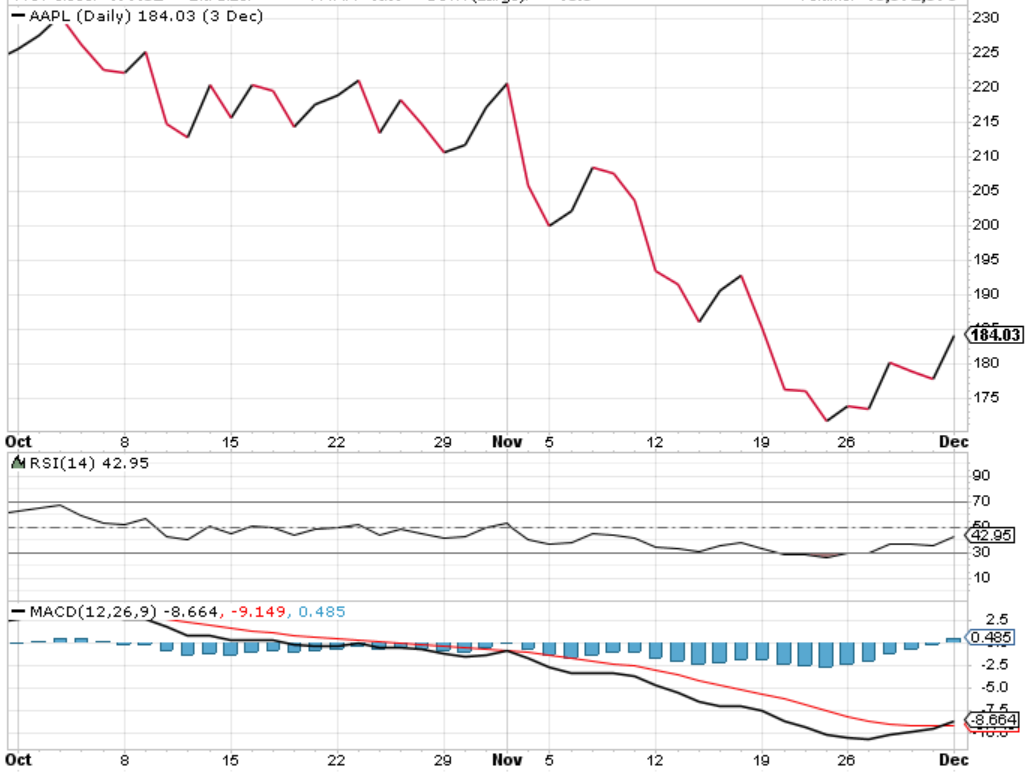You can see in the graph above that the MACD crossed below the centre line indicating a downtrend, but we would wait till Nov 2018 when the RSI breaches the 70 barriers but drops sharply afterwards. Here we have two indicators possibly telling us that the market is headed towards a bear run and sure enough, we can see that the closing price keeps declining till the end of November 2018. Thus we can go short on the stock in November and since we saw that the RSI indicator goes below 30 before rising again, we can exit the position.

### Difference between RSI indicator and MACD indicator

While we did mention that RSI indicators and MACD indicators have certain similarities, they have a different approach towards it. While RSI indicators consider the ratio of the gains and losses compared to the previous day, MACD is essentially a moving average of the price.

Thus, we should be careful and not expect that both the RSI and MACD will give the same signal at a particular point in time.

### Limitations of RSI

1. The fundamental property of RSI which states that a level above 70 is overbought can be proved wrong in a strong bull market where the company is progressing rapidly and posting good returns to its shareholders In this scenario the RSI can stay above 70 for a long time, which can be disastrous for short sellers. It is a similar case for a bear market where the RSI can stay below 30 and not rise above that level for an extended period of time.
2. The RSI can give false signals too. There are times when a divergence is indicated but the stock continues in its trend.
Thus, it is always said that we should use the RSI with one or more indicators which will give us a holistic view of the market and help us extract maximum information from the price action of a particular asset.

### Conclusion

In summary, once we know how to plot the RSI indicator, it is relatively easy to read it and form an opinion on the asset with the help of the RSI indicator graph. While a general level of 70 indicates overbought and 30 being oversold exists in the market, we have seen that it can be detrimental to rely on this bit of information entirely. Hence we try to use different strategies on the basis of RSI indicators to give us the necessary confidence to time the market profitably. We also saw that it is always better to use one or more indicator to help us in making more educated guesses in the market. The starter pack of Algorithmic Trading Strategies will help you create quantitative trading strategies using technical indicators which can adapt to live market conditions.